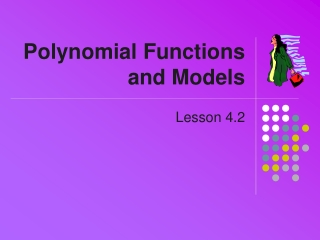DownloadDownload PresentationPolynomial Functions and Models

Polynomial Functions and Models

Télécharger la présentationPolynomial Functions and Models

- - - - - - - - - - - - - - - - - - - - - - - - - - - E N D - - - - - - - - - - - - - - - - - - - - - - - - - - -
Presentation Transcript

1. Polynomial Functions and Models Lesson 4.2

2. Review • General polynomial formula • a0, a1, … ,an are constant coefficients • n is the degree of the polynomial • Standard form is for descending powers of x • anxn is said to be the “leading term”

3. • Turning Points and Local Extrema • Turning point • A point (x, y) on the graph • Located where graph changes • from increasing • to decreasing • (or vice versa)

4. Family of Polynomials • Constant polynomial functions • f(x) = a • Linear polynomial functions • f(x) = m x + b • Quadratic polynomial functions • f(x) = a x2 + b x + c

5. Family of Polynomials • Cubic polynomial functions • f(x) = a x3 + b x2 + c x + d • Degree 3 polynomial • Quartic polynomial functions • f(x) = a x4 + b x3 + c x2+ d x + e • Degree 4 polynomial

6. Compare Long Run Behavior Consider the following graphs: • f(x) = x4 - 4x3 + 16x - 16 • g(x) = x4 - 4x3 - 4x2 +16x • h(x) = x4 + x3 - 8x2 - 12x • Graph these on the window -8 < x < 8       and      0 < y < 4000 • Decide how these functions are alike or different, based on the view of this graph

7. Compare Long Run Behavior • From this view, they appear very similar

8. Contrast Short Run Behavior • Now Change the window to be-5 < x < 5   and   -35 < y < 15 • How do the functions appear to be different from this view?

9. Contrast Short Run Behavior Differences? • Real zeros • Local extrema • Complexzeros • Note: The standard form of the polynomials does not give any clues as to this short run behavior of the polynomials:

10. Factored Form • Consider the following polynomial: • p(x) = (x - 2)(2x + 3)(x + 5) • What will the zeros be for this polynomial? • x = 2 • x = -3/2 • x = -5 • How do you know? • We see the product of two values • a * b = 0 We know that either a = 0 or b = 0 (or both)

11. Factored Form • Try factoring the original functions f(x), g(x), and h(x)  • (enter    factor(y1(x))  • what results do you get?

12. Local Max and Min • For now the only tools we have to find these values is by using the technology of our calculators:

13. Multiple Zeros • Given • We say the degree = n • With degree = n, the function can have up to n different real zeros • Sometimes the zeros are repeated, as seen in y1(x) and y3(x) below

14. Multiple Zeros • Look at your graphs of these functions, what happens at these zeros? • Odd power, odd number of duplicate roots => inflection point at root • Even power, even number of duplicate roots => tangent point at root

15. Linear Regression • Used in section previous lessons to find equation for a line of best fit • Other types of regression are available

16. Polynomial Regression • Consider the lobster catch (in millions of lbs.) in the last 30 some years • Enter into Data Matrix

17. Viewing the Data Points • Specify the plot F2, • X's from C1, Y's from C2 • View thegraph • Check Y= screen, use Zoom-Data

18. Polynomial Regression • Try for 4th degreepolynomial

19. Other Technology Tools • Excel will also do regression • Plot data as (x,y) ordered pairs • Right click on data series • Choose Add Trend Line

20. Other Technology Tools • Use dialog box to specify regression Try Others

21. Assignment • Lesson 4.2A • Page 247 • Exercises 1 – 41 odd • Lesson 4.2B • Page 249 • Exercises 43 – 57 odd